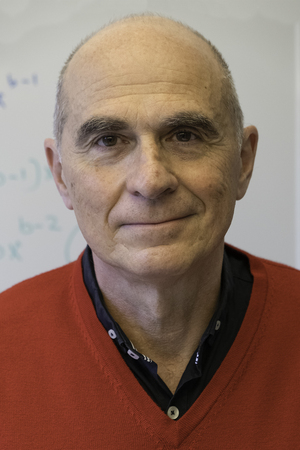## Institute of Mathematical Statistics and Actuarial Science# Prof. Dr. Ilya Molchanov

Professor of Probability Theory / Co-director of IMSV

Institute of Mathematical Statistics and Actuarial Science (IMSV)

Phone
+41 31 684 88 01
E-Mail
ilya.molchanov@unibe.ch
Office
-110
Alpeneggstrasse 22
CH-3012 Bern
Consultation Hour
• random sets (distributions, statistics, limit theorems, applications)
• stochastic geometry
• multivalued functions
• extreme values
• applications of stochastic geometry to
mathematical finance and econometrics
• multivariate stable laws and
stable laws on abstract structures
• point processes
• image processing
• mathematical morphology

### Some corrections to the second edition:

• Example 1.1.10: The conclusion is correct under additional assumption that K is connected (noticed by Samopriya Basu).
• Proposition 1.1.53: Here the family K_0 should also contain unions of all relatively compact sets from the base of topology, e.g. union of balls in Euclidean space. (Correction provided by Daniel Hug).
• Theorem 1.4.25 was cited from Teran . The author informed me that later that M.Dumav and M.Stinchcombe (Proc. Amer. Math. Soc., 2016) provided a counterexample. Still it is not clear what is a gap in the proof by Teran .
• Theorem 3.1.31 was cited from Teran . The author informed that this result holds only assuming that the dual space is separable.

### Minor misprints

• p.468, line 2: In the second line of Example 5.1.29 x should be replaced by t.

### Membership in scientific societies

• Member: Bernoulli Socienty, Institute of Mathematical Statistics, Swiss Mathematical Society, London Mathematical Society
• Fellow of the Royal Society of Edinburgh